## Reading and writing large numbers in words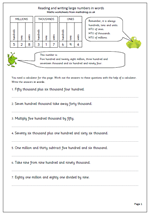A calculator is needed for this worksheet, but this does not make it any easier. First of all the calculation is written in words rather than numbers and needs to be read correctly in order for it to be keyed into the calculator correctly. The calculator comes up with the answer in digits and this has to be converted to words in the answer.

Many children, and indeed adults, find it very difficult to read large numbers correctly. The solution is to section the digits off into groups of three, starting with the units.

The first group of three is read as hundreds, tens and units.

The second group of three is read as hundreds, tens and units of thousands.

The third group of three is read as hundreds, tens and units of millions.

So 528528528

is five hundred and twenty eight million, five hundred and twenty eight thousand and five hundred and twenty eight. Easy!

This page can be found in the Year 6 Understanding Number category.

Read and write large numbers in words

## Year 3 mental Arithmetic: Sets 23 and 24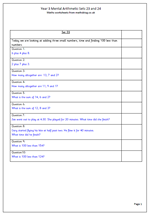Here we have another two sets of ten mental arithmetic questions for Year 3. This week the questions concentrate on adding three small numbers, time and finding 100 less than a number.

When adding three digits mentally it is usually easier to do it in the order that the question is spoken because it is easier to retain the three correct numbers ‘in your head’. However, still look for pairs of numbers which make multiples of 10 as this makes the question much easier to answer.

Perhaps a point to raise here is that each of the questions should be read out loud at least twice, if not more, to gice children the best chance of answering correctly.

By the end of year 3 children should be quite confident with time questions and adding minutes to a set time.

Year 3_Mental Arithmetic_Sets 23 and 24

## Resource of the Week: One minute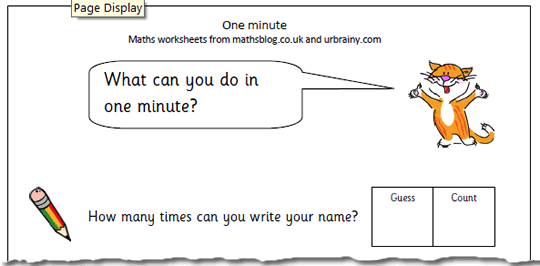When learning about time most people think about reading clock faces, but there is much more than this with young children. Getting a clear concept of amount of time is important for young children. In other words, what is it realistic to be able to done in one second, one minute or one hour? This page challenges children to complete a number of tasks in one minute, such as how tall a tower can be built using blocks/lego etc.

An important part of this is to make sure that they make an estimate/guess before starting which shows how realistic they are about time. This resource can be found in our Year 2 measurement section.

One minute

## KS2 Maths Paper 2010 Question 12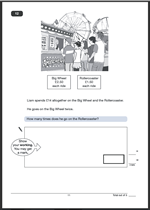This is a typical SAT question, which many children fail to get correct. The main reason for this is that it is in three parts and many children cannot progress through a step by step approach, wanting to find the answer in one easy move. The second reason is that it involves dividing by a decimal fraction at the end.

The question asks how many times Liam goes on the Rollercoaster, with each ride costing £1.50.

Begin by finding how much two goes on the big wheel costs as this will show how much he has spent before going on the Rollercoaster.

That’s 2 x £2.50 which is £5.

Now to find how much he has left to spend. Subtract the £5 from the total spent.
That’s £14 – £5 = £9
Then work out how many times Liam could go on the Rollercoaster with £9. Probably the easiest way to do this is to work out that two rides would cost £3, so 6 rides would cost £9. Now, whilst the calculation might have been done mentally it is important to write down what was done.

Two marks for a correct answer, but one mark can be given if the correct method is shown.

Question 12 from SATS Paper A 2010

Question 12 answer and suggested method.

## Year 3 Mental Arithmetic: Sets 21 and 22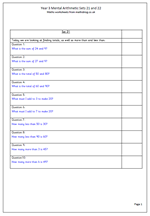Here we have the next two sets of questions in the Year 3 Mental Arithmetic series. This week the questions concentrate on using the vocabulary of addition and subtraction, including:

What is the sum of….?

What is the total of….?

What must I add to …. to make ….?

How many more than … is …?

How many less than … is …?

It is very useful to spend some time on asking children how they approach these questions, ‘in their heads’. For example:

‘What must I add to 7 to make 20?’ can be approached in several ways.

One way is to add on 3 to make 10 and then 10 more to make 20.

Another way is to add 10 and then count on 3.

A further method would be to take 10 from 20 and then count back 3 more.

By asking children to explain their methods it makes them think about what they have done and they will often see that there is a more efficient method than the one they used. The ultimate aim of this is to develop quick and efficient methods which produce correct answers.

Year 3 Mental Arithmetic: Sets_21 and 22

## Multiply the Egyptian way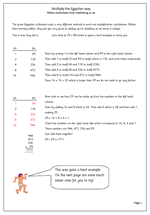Sometimes it can help children’s understanding of maths and numbers if they are shown a completely different way of approaching a calculation. In Year 6 most children will be familiar with the standard written method of multiplication, but this method is far from the only one which can be used. It just happens to be quite an efficient method which is relatively easy to use.

The great Egyptian civilisation used a very different method to work out multiplication calculations. Rather than learning tables, they just got very good at adding up pairs of the same number (or doubling as we know it today). One of the reasons for this is that they did not have multiplication ‘tables’.

The method is quite long, but nevertheless quite easy if you are good at addition and it is explained in detail on the first worksheet.

Egyptian multiplication

## Resource of the Week: Division with remainders as fractions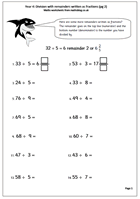This week we are looking at worksheets where the remainders from division are written as fractions. Children need a good knowledge of tables to work out the division ‘in their heads’ and this is probably best suited to Year 4, aged8/9+ years old.

Children will be used to the concept of a remainder when dividing but this goes one step further, removing the remainder altogether. When writing the answer as a fraction the remainder goes on the top line (numerator) and the bottom number (denominator) is the number you have divided by.

This is a much neater finish to the question as the whole number is divided completely, with no messy remainders. Some of the fractions could be cancelled down to their simplest form, although this is not essential at this stage. There are several similar pages to be found in our Year 4 Calculating category.

Division with fraction remainders (pg 2)

## KS2 Maths Paper 2010 Questions 10 and 11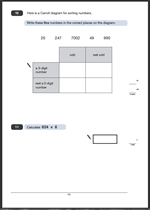Carroll diagrams are a favourite with the SAT paper writers and it is no surprise to find one in the 2010 SAT paper 1. There is nothing tricky about this and the best approach is to take each number in turn, look at its properties and decide where it should go.
Look at 25. Is it odd? Yes, so it must go in the first column. Is it a 3-digit number or not a 3-digit number? It is a 2-digit number so it will go in the second row. Continue with the other numbers in turn.

Two marks are given for a completely correct answer and one mark can be gained if only one number is placed incorrectly, or is missing.

For question 11 I would recommend writing this out as a multiplication in the standard way. Watch out for children who forget to write the answer in the box after completing the calculation, although they should still be awarded the mark, if the marker follows the guidelines.

Questions 10 and 11 from 2010 Paper A

Questions 10 and 11 from 2010 Paper A answers

## Year 3 Mental Arithmetic: Sets 19 and 20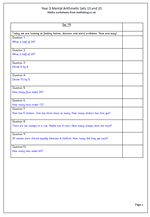Today I have published another two sets of 10 mental arithmetic questions suitable for Year 3 children (7 to 8 years old). This week I have concentrated on;

a. finding halves of even 2-digit numbers

b. dividing by 4 and 5

c. simple word problems.

Year 3 is an important stage in children’s mathematical development as there is a move away from the concrete to the more abstract. It is a year when it is expected that tables are starting to be learnt, but it is vital that they understand what they mean. Questions involving tables can be asked in a wide variety of ways, including the equivalent division calculation.

Don’t forget that these questions can be answered on paper, by calling out, or by using number cards or number fans. Each way has its own advantages.

All the mental arithmetic papers can be found in the ‘Know Number Facts’ category of the Year 3 worksheets.

Year 3 mental arithmetic sets 19 and 20

## Year 4 decimals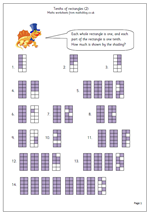One of the best ways to introduce decimals is by working with money which shows the idea of tenths (10p) and hundredths (1p). Another good way is to use shading of shapes and this is the second page which does this. A series of rectangles is shown, each divided into ten equal parts: each part being one tenth. This is a very clear illustration of what tenths are and children should find it relatively easy to write down how much is shown: this could also be used on a whiteboard as a whole class activity at the start of the lesson.

It could also be used to show that 1/10 is the same as 0.1, 2/10 the same as 0.2 etc.

This is the second page of Tenths of Rectangles and can be found in our year 4 Counting and Numbers category.

Tenths of rectangles (2)